# Seaborn 統計關係

X與Y軸關係散佈圖

``````import pandas as pd
import seaborn as sns

csv_url = 'https://raw.githubusercontent.com/aicourse-org/dataset/main/titanic/titanic.csv'

df.sample(3)``````
survived pclass name sex age sibsp parch ticket fare cabin embarked
328 1 3 Goldsmith, Mrs. Frank John (Emily Alice Brown) female 31.0 1 1 363291 20.525 NaN S
365 0 3 Adahl, Mr. Mauritz Nils Martin male 30.0 0 0 C 7076 7.250 NaN S
372 0 3 Beavan, Mr. William Thomas male 19.0 0 0 323951 8.050 NaN S

## X軸 與 Y軸

``ax = sns.relplot(data=df, x='age', y='fare')``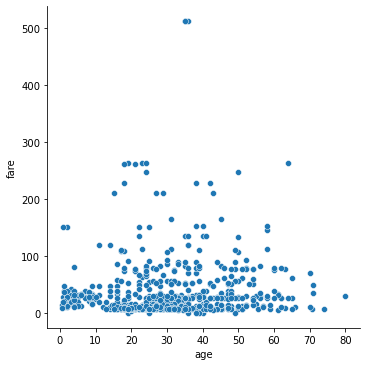``df_m = df[df['fare']<300]``
``ax = sns.relplot(data=df_m, x='age', y='fare')``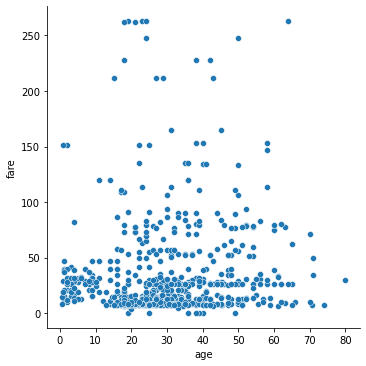## 新增圖層

``ax = sns.relplot(data=df_m, x='age', y='fare', style='sex')``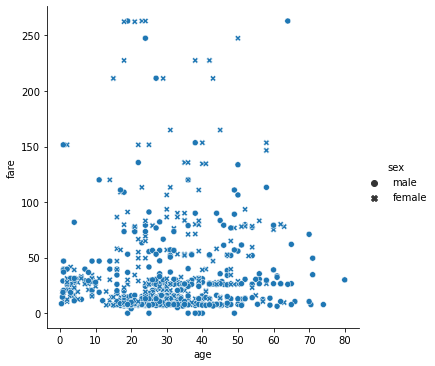``ax = sns.relplot(data=df_m, x='age', y='fare', col='sex')``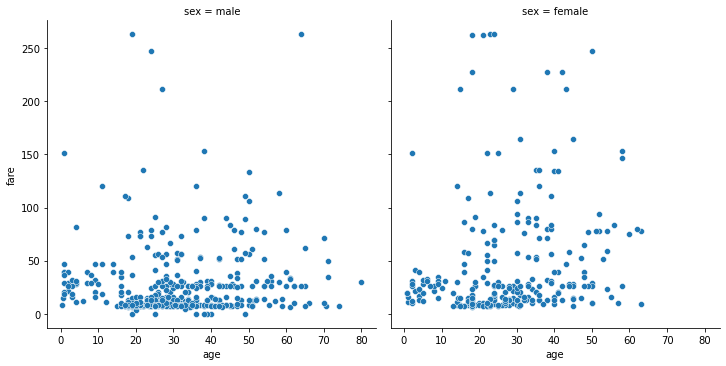``ax = sns.relplot(data=df_m, x='age', y='fare', hue='pclass')``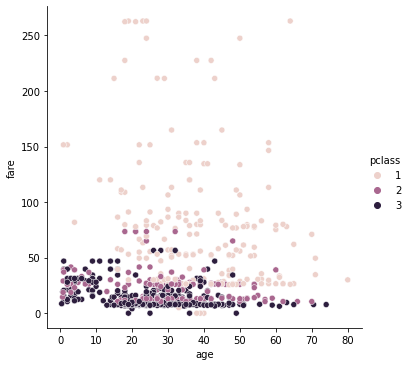``ax = sns.relplot(data=df_m, x='age', y='fare', hue='survived')``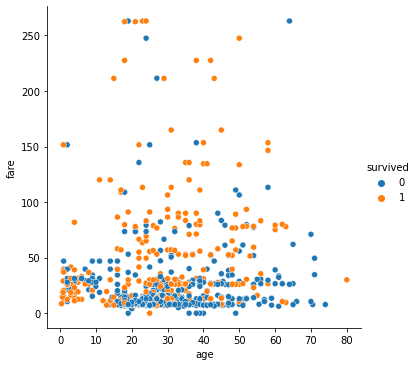``ax = sns.relplot(data=df_m, x='age', y='fare', col='pclass', hue='survived')``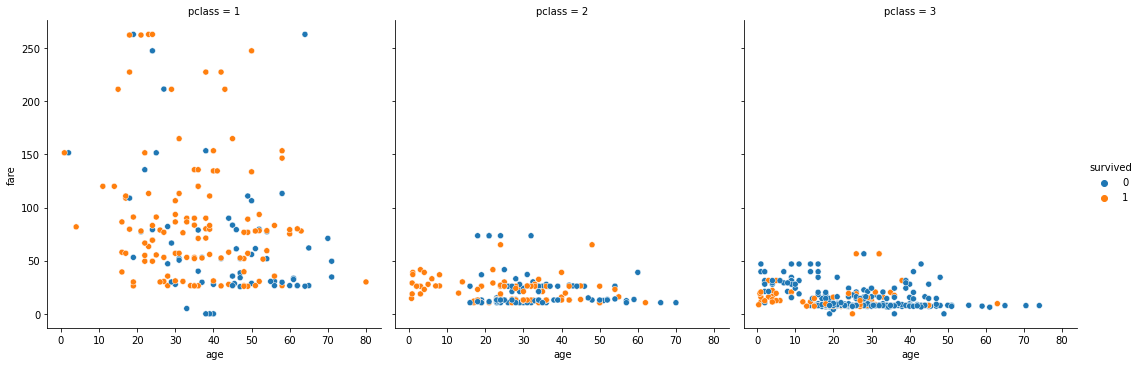``ax = sns.relplot(data=df_m, x='age', y='fare', col='pclass', hue='survived', size='sex')``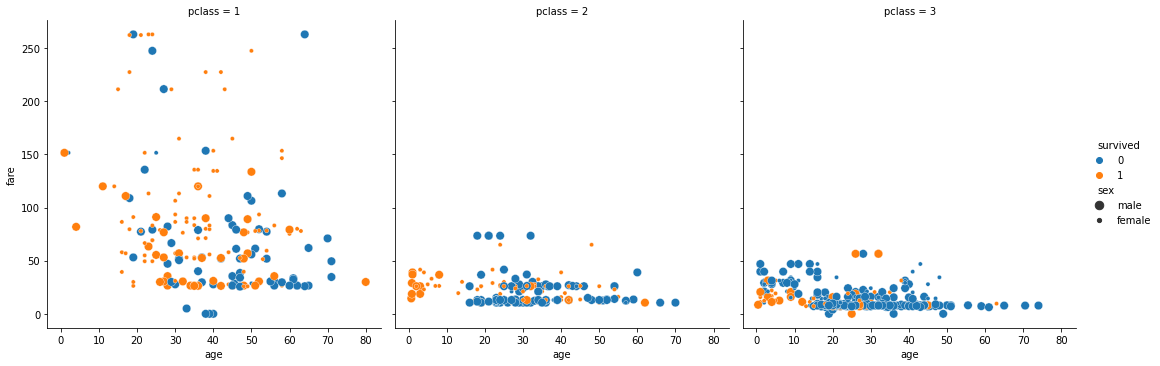v1.0